# Island Width Evolution

Let us write the generalized Rutherford island width evolution equation, (13.7), in the form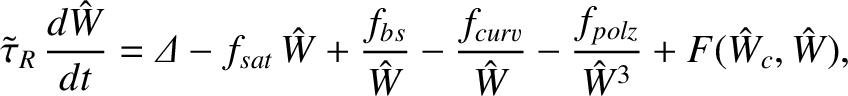(13.63)

where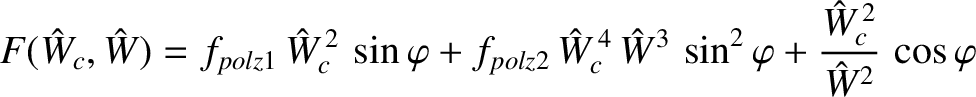(13.64)

represents the three additional terms that appear on the right-hand side of the equation when an island chain interacts with a static resonant magnetic perturbation.

First, let us consider a rotating magnetic island chain. Given that the island width evolves on a much longer timescale than the island phase (see Table 13.2), it is a good approximation to replace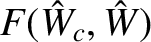by its period average in the generalized Rutherford equation [9,10]. [See Equation (13.49).] Now,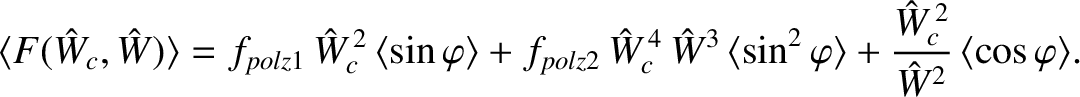(13.65)

However, to lowest order,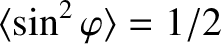,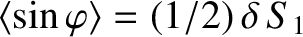, and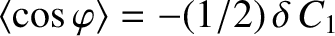. [See Equations (13.51) and (13.52).] Thus, we obtain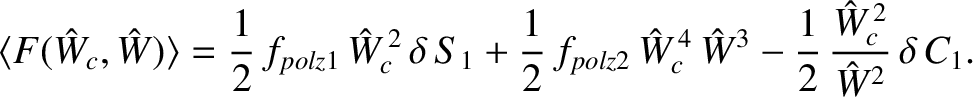(13.66)

As is clear from Table 13.1 and Equations (13.29)–(13.31), the first two terms on the right-hand side of the previous equation, which are ion polarization terms that arise from the modified ion flow in the immediate vicinity of the island chain when the chain is subject to an electromagnetic locking torque, are destabilizing (i.e., positive). On the other hand, the third term, which is due to the direct interaction between the island chain and the static resonant magnetic perturbation, is stabilizing (i.e., negative).

If we use the data given in Tables 13.1 and 13.2 to estimate the sizes of the three terms on the right-hand side of the previous equation in a tokamak fusion reactor then we discover that the first two terms are completely negligible compared with the third. In other words, the polarization terms associated with the modified ion flow in the vicinity of the island chain are much smaller than the term due to the direct interaction between the island chain and the static resonant magnetic perturbation. In this limit, we can use Equation (13.29) to write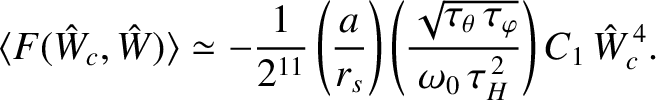(13.67)

Thus, we conclude that the interaction between a rotating island chain and a static magnetic perturbation gives rise to a stabilizing term in the chain's Rutherford equation that is independent of the island width, and scales as the square of the current flowing in the magnetic field-coil that generates the perturbation. [See Equation (13.3).] Making use of the data given in Table 13.2, we deduce that the stabilizing term takes the form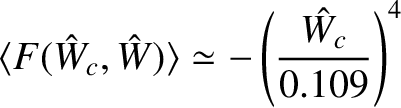(13.68)

in a low-field tokamak fusion reactor (with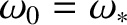), and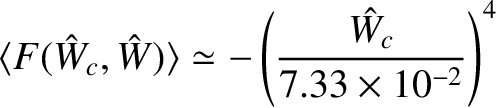(13.69)

in a high-field tokamak fusion reactor. Of course, these estimates are only valid as long as the locking criteria (13.53) and (13.54) are not satisfied. Equations (13.53), (13.54), (13.68), and (13.69) suggest that the stabilizing effect of the interaction of a rotating magnetic island chain with a static resonant magnetic perturbation could completely stabilize a relatively narrow island chain, but would not be able to completely stabilize a wide island chain, because the chain would lock to the perturbation before complete stabilization was acheived. The complete stabilization of a narrow rotating magnetic island chain by a static resonant magnetic perturbation has been observed experimentally .

Let us now consider a locked magnetic island chain. Equations (13.59), (13.60), and (13.64) yield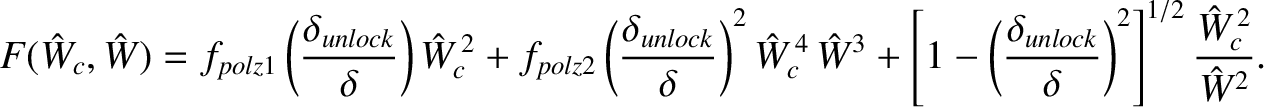(13.70)

Of course, the previous equation is only valid when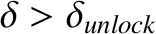(i.e., provided that the island chain remains locked to the static resonant magnetic perturbation). Assuming that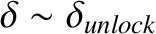,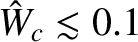, and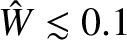, it is clear from the data given in Table 13.1 that the first two terms on the right-hand side of the previous equation are completely negligible compared to the third. In other words, just as is the case for a rotating island chain, the polarization terms in the Rutherford equation associated with the modified ion flow in the vicinity of the island chain are much smaller than the term due to the direct interaction between the island chain and the static resonant magnetic perturbation. In this case, we can write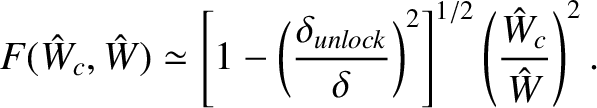(13.71)

Thus, it is clear that the destabilizing effect of a static resonant magnetic perturbation on locked island chain can be significant, especially in the limit in which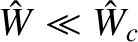. This destabilizing effect has been observed experimentally .

Finally, experiments have been performed in which the rotation frequency of a magnetic island chain in a tokamak plasma is controlled by locking the chain to a rotating resonant magnetic perturbation . More ambitious experiments have been performed in which the helical phase of a rotating resonant magnetic perturbation is actively controlled in such a manner that a magnetic island chain in a tokamak plasma is held in a permanent stabilizing phase relation with respect to the perturbation [19,21]. Unfortunately, neither of these control schemes is practical in a tokamak fusion reactor because the magnetic field-coils that generate the rotating magnetic perturbation would have to be placed inside the vacuum vessel (otherwise the oscillating magnetic field generated by the coils would be shielded from the plasma by eddy currents excited in the vessel), where they would be unacceptably vulnerable to neutrons produced by fusion reactions within the plasma, as well as large electromagnetic forces generated by plasma disruptions.